Win up to 100% scholarship on Aakash BYJU'S JEE/NEET courses with ABNAT Win up to 100% scholarship on Aakash BYJU'S JEE/NEET courses with ABNAT

# JEE Main 2021 February 25 – Shift 2 Chemistry Question Paper with Solutions

Candidates can find the JEE Main 2021 February 25 Shift 2 Chemistry paper with solutions here. All the questions are solved by our subject experts in a detailed manner. Watch the video below to get familiar with the JEE Main Chemistry exam pattern, the marking scheme and the type of questions that are asked in the exam. Stay tuned, while we are in the process of providing solutions for JEE Main 2021 February 25 Shift 2 Chemistry question paper.

### JEE Main 2021 February 25 – Shift 2 Chemistry Question Paper with Solutions

JEE Main 2021 25th February Shift 2 Chemistry Question Paper

SECTION A

Question 1. Given below are two statements:

Statement I: The identification of Ni2+ is carried out by dimethylglyoxime in the presence of NH4OH

Statement II: The dimethylglyoxime is a bidentate neutral ligand.

In the light of the above statements, choose the correct answer from the options given below:

a. Both statement I and statement II are true
b. Both statement I and statement II are false
c. Statement I is false but statement II is true
d. Statement I is true but statement II is false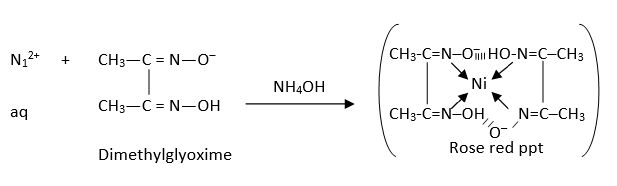Dimethylglyoxime is a negative bidentate legend.

Question 2. Carbylamine test is used to detect the presence of a primary amino group in an organic compound. Which of the following compounds is formed when this test is performed with aniline?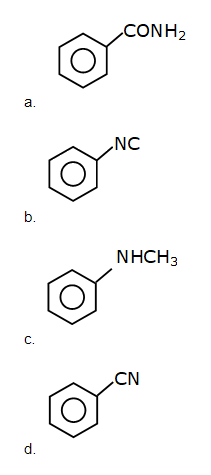Question 3. The correct order of bond dissociation enthalpy of halogen is:

a. F2>Cl2>Br2>I2
b. Cl2>F2>Br2>I2
c. Cl2>Br2>F2>I2
d. I2>Br2>Cl2>F2

Fact based

F2 has F — F, F2 involves repulsion of non-bonding electrons & more over its size is small and hence due to high repulsion its bond dissociation energy is very low.

Question 4. Which one of the following statements is FALSE for hydrophilic sols?

a. These sols are reversible in nature
b. The sols cannot be easily coagulated
c. They do not require electrolytes for stability
d. Their viscosity is of the order of that of H2O

Fact based

Question 5. Water does not produce CO on reacting with

a. C3H8
b. C
c. CH4
d. CO2

H2O + CO2 H2CO3

Question 6. What is ‘X’ in the given reaction?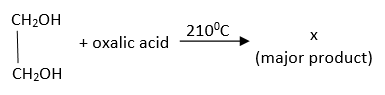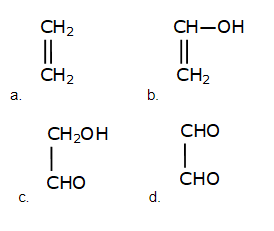Question 7. If which of the following orders the given complex ions are arranged correctly with respect to their decreasing spin only magnetic moment?

(i) [FeF6]3-

(ii) [Co(NH3)6]3+

(iii) [NiCl4]2-

(iv) [Cu(NH3)4]2+

a. (ii)>(i)>(iii)>(iv)
b. (iii)>(iv)>(ii)>(i)
c. (ii)>(iii)>(i)>(iv)
d. (i)>(iii)>(iv)>(ii)

[FeF6]3-: Fe3+ 3d5 → 5-unpaired electrons as F is weak field legend

[Co(NH3)6]3+: Co3+ 3d6→ No-unpaired electron as NH3 is strong field light and causes pairing

[NiCl4]2-: Ni2+ 3d8 → 2-unpaired electrons

[Cu(NH3)4]2+: Cu2+ 3d9 → 1-unpaired electrons

Question 8. The major product of the following reaction: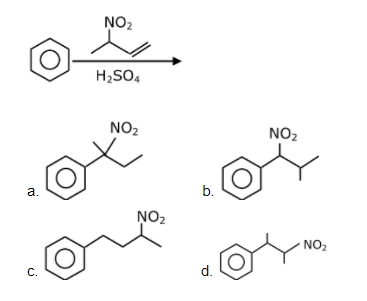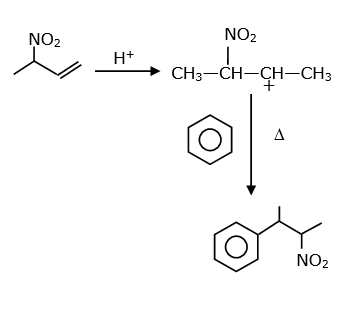Question 9: The correct sequence of reagents used in the preparation of 4-bromo-2-nitroethyl benzene from benzene is:

a. CH3COCl/AlCl3, Br2/AlBr3, HNO3/H2SO4, Zn/HCl
b. CH3COCl/AlCl3, Zn-Hg/HCl, Br2/AlBr3, HNO3/H2SO4
c. Br2/AlBr3, CH3COCl/AlCl3, HNO3/H2SO4, Zn/HCl
d. HNO3/H2SO4, Br2/AlCl3, CH3COCl/AlCl3, Zn-Hg/HClQuestion 10. The major components of German Silver are:

a. Cu, Zn and Mg
b. Ge, Cu and Ag
c. Zn, Ni and Ag
d. Cu, Zn and Ni

Fact

German silver is alloy which does not have silver.

Cu-50%; Ni-30%; Zn-20%

Question 11. The method used for the purification of Indium is:

a. Van Arkel method
b. Vapour phase refining
c. Zone refining
d. Liquation

Fact

Ga, In, Si, Ge are refined by zone refining or vacuum refining.

Question 12. Which of the following is correct structure of α-anomer of maltose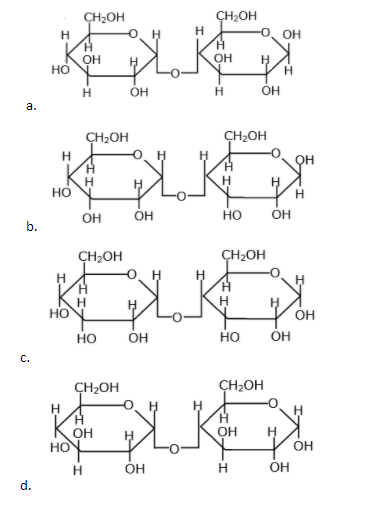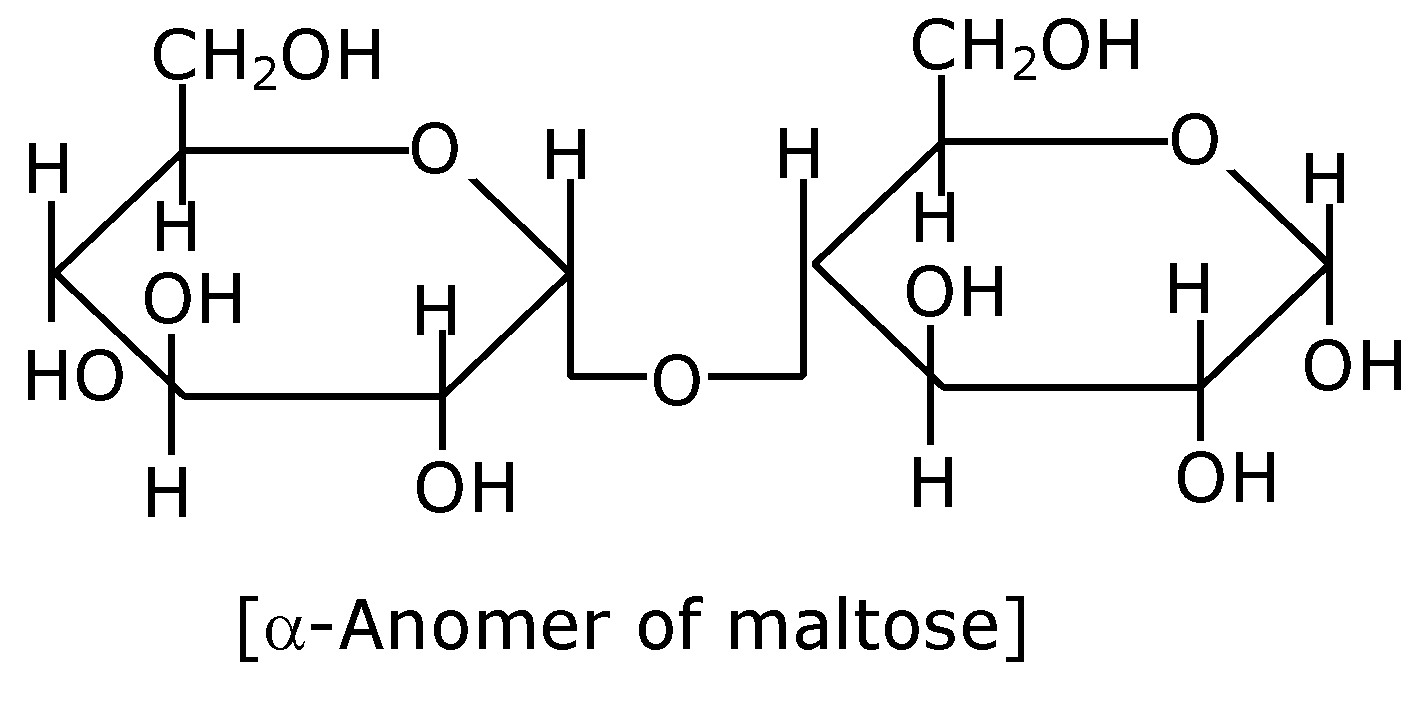Question 13. The major product of the following reaction is:a. CH3CH2CH2CHO
b. CH3CH2CH=CH–CHO
c. CH3CH2CH2CH2CHO
d.Question 14. The correct order of acid character of the following compounds is:a. II>III>IV>I
b. III>II>I>IV
c. IV>III>II>I
d. I>II>III>IV

Acidity of carboxylic acid α-R>-H>-I

1 / α + R > + H > + I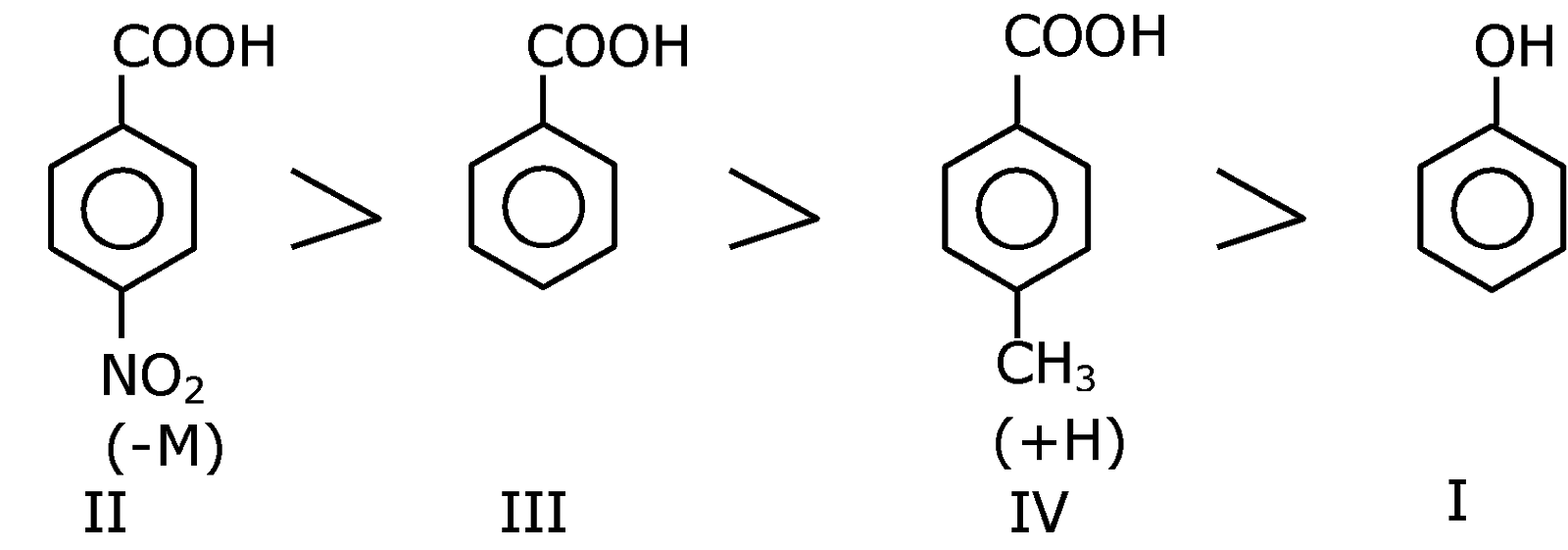Question 15. Which among the following species has unequal bond lengths?

a. XeF4
b. SiF4
c. BF4
d. SF4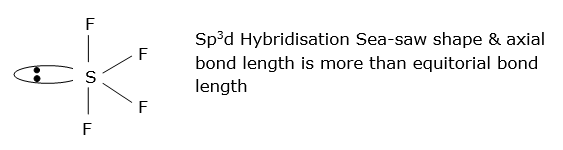Question 16. Given below are two statements:

Statement I: α and β forms of sulphur can change reversibly between themselves with slow heating or slow cooling.

Statement II: At room temperature the stable crystalline form of sulphur is monoclinic sulphur.

In the light of the above statements, choose the correct answer from the options given below.

a. Both statement I and statement II are false
b. Statement I is true but statement II is false
c. Both statement I and statement II are true
d. Statement I is false but statement II is true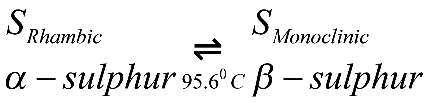Question 17. Correct statement about the given chemical reaction is: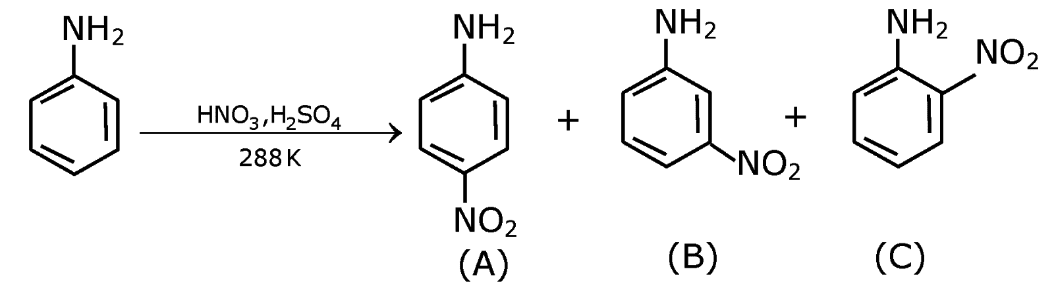a. Reaction is possible and compound (A) will be a major product.
b. The reaction will form a sulphonated product instead of nitration.
c. NH2 group is ortho and para directive, so product (B) is not possible.
d. Reaction is possible and compound (B) will be the major product.Question 18. Which of the following compound is added to the sodium extract before addition of silver nitrate for testing of halogens?

a. Nitric acid
b. Sodium hydroxide
c. Hydrochloric acid
d. Ammonia

NaCN + HNO3 → NaNO3 + HCN ↑

Na2S + HNO3 → NaNO3 + H2S ↑

Nitric acid decomposes NaCN & Na2S, else they precipitate in test & misguide the resolve.

Question 19: Given below are two statements:

Statement I: The pH of rain water is normally ~5.6.

Statement II: If the pH of rain water drops below 5.6, it is called acid rain.

In the light of the above statements, choose the correct answer from the option given below.

a. Statement I is false but Statement II is true
b. Both statement I and statement II are true
c. Both statement I and statement II are false
d. Statement I is true but statement II is false

Both statements are correct

Question 20. The solubility of Ca(OH)2 in water is:

[Given: The solubility product of Ca(OH)2 in water = 5.5×10-6]

a. 1.11 ×10-6
b. 1.77 ×10-6
c. 1.77×10-2
d. 1.11×10-2

Ca(OH)2 ⇌ Ca+2 + 2OH

s (2s + 10-7)

s(2s+10-7)2 = 55×10-7

4s3 = 55×10-7

s3 = 5500 / 4 × 10-9

$$\begin{array}{l}s = \left ( \frac{2250}{2} \right )^{\frac{1}{3}}\times 10^{-3}\end{array}$$

s = (1125)× 10-3

s = 1.11 × 10-2

SECTION B

Question 1. If a compound AB dissociates to the extent of 75% in an aqueous solution, the molality of the solution which shows a 2.5 K rise in the boiling point of the solution is ______molal.

(Rounded-off to the nearest integer)

[Kb =0.52 K kg mol-1]

AB → A+ + B

1 – α α α

α = 3 / 4

N = 2

i = [1+(2-1)α]

2.5 = [1+(2-1)3/4] × 0.52 × m

$$\begin{array}{l}m = \frac{2.5}{0.52\times \frac{7}{4}} = \frac{10}{3.64} = 2.747\end{array}$$

m= 2.747≈ 3 mol/kg

Question 2. The spin only magnetic moment of a divalent ion in aqueous solution (atomic number 29) is ____BM.

29Cu+2 → [Ar]183d9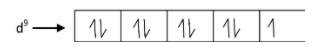No. of unpaired e = 1

Magnetic moment = μ = √n(n + 2)

μ = √(1)(1 + 2) = √3B.M.

= 1.73 B.M

Question 3. The number of compound/s given below which contain/s —COOH group is ______

a. Sulphanilic acid
b. Picric acid
c. Aspirin
d. Ascorbic acid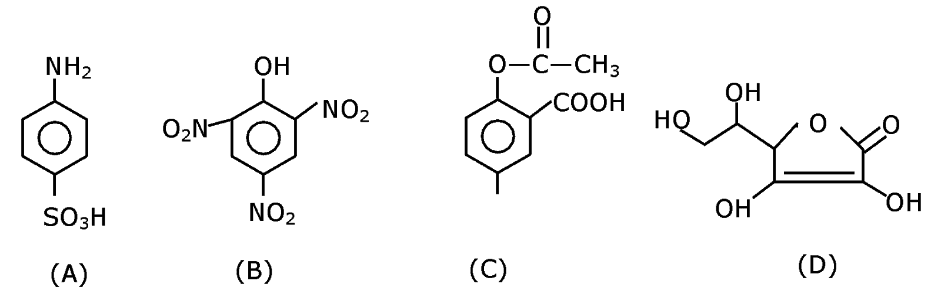Question 4. The unit cell of copper corresponds to a face centered cube of edge length 3.596 Å with one copper atom at each lattice point. The calculated density of copper in kg/m3 is ______.

[Molar mass of Cu : 63.54 g; Avogadro number = 6.022×1023]

a = 3.596 Å

$$\begin{array}{l}d = \frac{Z\times GMM}{N_{A}\times a^{3}}\end{array}$$
$$\begin{array}{l}d = \frac{Z4\times63.54\times 10^{-3}}{6.022\times 10^{23}\times (3.956\times 10^{-10})^{3}}\end{array}$$

d = 0.9076 × 104 = 9076.2 kg/m3

Question 5. Consider titration of NaOH solution versus 1.25 M oxalic acid solution. At the end point following burette readings were obtained.

(i) 4.5 mL (ii) 4.5 mL (iii) 4.4 mL (iv) 4.4 mL (v) 4.4 mL

If the volume of oxalic acid taken was 10.0 ml. then the molarity of the NaOH solution is ____M. (Rounded-off to the nearest integer)

Eq. of NaOH = Eq. of oxalic acid

[NaOH] × 1 × 4.4 = 5/4 × 2 ×10

[NaOH] = 100 / 4 × 4.4 = 25 / 4.4 = 5.68

Nearest integer = 6 M

Question 6. Electromagnetic radiation of wavelength 663 nm is just sufficient to ionize the atom of metal A. The ionization energy of metal A in kJ mol—1 is____. (Rounded off to the nearest integer)

[h=6.63×10-34Js, c = 3.00×108ms-1, NA=6.02×1023 mol-1]

Energy required to ionize an atom of metal ‘A’ = hc / λ = hc / 663 nm for 1 mole atoms of ‘A’

Total energy required = NA × hc / λ

=

$$\begin{array}{l}\frac{6.023\times10^{23} \times6.63 \times10^{-34} \times3 \times10^{8}}{663\times 10^{-9}}\end{array}$$

= 6.023 × 3 × 1023-34+8+7

= 18.04 × 104 J/mol

= 180.4 kJ/mol

Nearest Integer = 180 kJ/Mol.

Question 7. The rate constant of a reaction increases by five times on increasing temperature from 270 C to 520C. The value of activation energy in kJ mol-1 is ______.

(Rounded off to the nearest integer)

[R=8.314 J K-1 mol-1]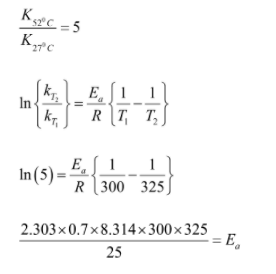Ea = 51524.96 J/mol

Ea = 51.524 kJ/mol

52 Ans.

Question 8. Copper reduces into NO and NO2 depending upon the concentration of HNO3 in solution. (Assuming fixed [Cu2+] and PNO=PNO2), the HNO3 concentration at which the thermodynamic tendency for reduction of into NO and NO2 by copper is same is 10x M.

The value of 2x is ______. (Rounded-off to the nearest integer)

[Given:
$$\begin{array}{l}E_{Cu^{2+} / Cu}^{0} = 0.34V, E_{No_{3}^{-} / NO}^{0} = 0.96V, E_{No_{3}^{-} / NO_{2}}^{0} = 0.79V\end{array}$$
and at 298 K,
$$\begin{array}{l}\frac{RT}{F}(2.303)= 0.059\end{array}$$
]

Anode

Cu(s) → Cu+2 + 2e-

Cathode (1)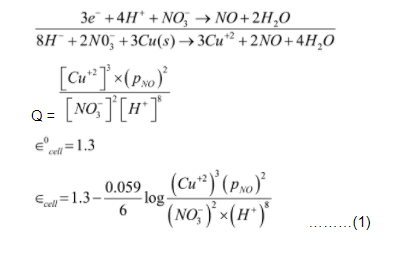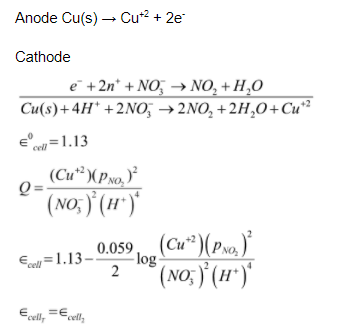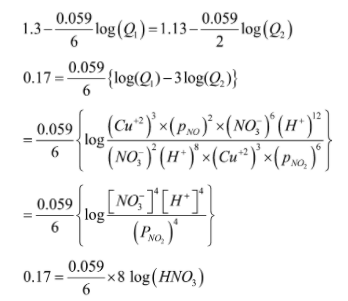log (HNO3) = 2.16

[HNO3] = 102.16 = 10x

x = 2.16 ⇒ 2x = 4.32 ≈ 4

Question 9. Five moles of an ideal gas at 293 K is expanded isothermally from an initial pressure of 2.1 MPa to 1.3 MPa against a constant external 4.3 MPa. The heat transferred in this process is ____kJ mol-1. (Rounded-off of the nearest integer)

[Use R = 8.314 J mol-1 K-1]

Moles (n) = 5

T = 293 K

Process = Iso T. → Irreversible

Pini = 2.1 M Pa

Pt = 1.3 M Pa

Pext = 4.3 mPa

Work = – Pext Δv

$$\begin{array}{l}= -4.3\times \left ( \frac{5\times 293R }{1.3}-\frac{5\times293 }{2.1} \right )\end{array}$$
$$\begin{array}{l}= -5\times293 \times8.314 \times43 \left ( \frac{1}{13}-\frac{1}{21} \right )\end{array}$$
$$\begin{array}{l}= \frac{5\times293 \times8.314 \times43\times 8 }{21 \times 13}\end{array}$$

= -15347.7049 J

= – 15.34 kJ

Isothermal process, so ΔU = 0

w = – Q

Q = 15.34 kJ / mol

Question 10. Among the following, the number of metal/s which can be used as electrodes in the photoelectric cell is _____(Integer answer).

a. Li

b. Na

c. Rb

d. Cs